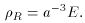### 3. A BRIEF HISTORY OF THE UNIVERSE

One of the successes of the hot Big Bang model is its prediction of the light elements. These predictions are verified by observations of the structure and composition of the oldest stars, quasars, and other quasi-stellar remnants (e.g., QSOs) [18, 19, 20, 21]. The process by which the elements form is referred to as nucleosynthesis.

The Hot Big-Bang model predicts a universe that will go through several stages of thermal evolution. As the universe expands adiabatically, the temperature cools, scaling asHereis the ratio of specific heats and is equal to 4/3 for a radiation dominated universe . This relation is manifest, since the temperature is equivalent to the energy density divided by the volume (in natural units= c = k = 1). Moreover, the radiation energy density is redshifted by an additional factor of 1/a(t) since the Hubble expansion stretches the wavelength, which is inversely proportional to the energy:andSetting the Boltzman constant to unity,(41)

This can also be understood using the DeBroglie wavelength of the photon (for radiation) . The wavelength is inversely proportional to the energy in natural units. This raises the issue of a possible factor of redshifting for the DeBroglie wavelength of a massive particle. For a particle the simple relation E = pc does not hold; thus, the velocity of the particle can decrease to preserve its wavelength. This redshifting is analogous to that of equation (3) in the first section and gives an alternative explanation of particles in motion settling into the cosmic rest frame. This also explains why one might expect to find primarily non-relativistic (cold) matter, which just means particles traveling at speeds much less than c.

From relation (41) for the temperature, one has a quantitative way to find critical temperature scales in the evolution of the universe. For a given value of the curvature the relation between the scale factor and time can yield an expression between temperature and time. For example, in a matter dominated, flat universe,which was derived earlier. In thermal physics one is usually interested in thermal equilibrium. This consideration is accounted for by the condition,This relations shows that the reaction rate,, must be much greater than the rate at which the universe expands for thermal equilibrium to be reached.is related to the cross-section of the given particle interaction bywhereis the cross-section, |v| is the relative speed, and n is the number density of the species (14)

From equation (41), one can see that the universe began as a point of infinite temperature and zero size. This is a singular point for the history of the universe and the standard Big Bang model (General Relativity) breaks down at this singularity (15) However, after the Planck time (10-43) s one can follow the evolution using the concepts of thermal physics and particle theory (16)

In the earliest times following the Planck epoch, all matter existed as free quarks and leptons. The existence of free quarks (known as asymptotic freedom) is made possible by the high energy (temperature) during the early moments of the Big Bang. The universe cools, as indicated by (41), and the quarks begin to combine under the action of the strong force to form nucleons. This phase of formation is referred to as Baryogenesis, because the baryons (e.g., protons and neutrons) are created for the first time. The expansion continues to allow leptons, such as electrons, to interact with nucleons to form atoms. At this point, referred to as recombination, the photons in the universe are free to travel with virtually no interactions. For example, hydrogen is the most abundant element to form in nucleosynthesis and at the time of decoupling the temperature of the photons has dropped to around 3000 K. This corresponds to less than 13.6 eV, the energy needed to ionize the atoms. Therefore, there are no longer free electrons to interact with the photons and in fact the energy of a photon (around .26 eV, at this temperature) is so low that it can not interact with the atoms. In this way the photons have effectively decoupled from matter and travel through the universe as the cosmic background discussed in Part II. A brief summary of the most significant events are encapsulated below,

• 10-4 seconds: Baryogenesis occurs, quarks condense under strong interaction to form nucleons (e.g., Protons and Neutrons)

• 1 second: Nucleosynthesis occurs, universe cools enough (photon energies ~ 1 MeV) for light nuclei to form (e.g., deuterons, alpha particles).

• 104 years: Radiation density becomes equal to matter density, since the radiation density has extra factor of a-1 due to red-shifting. Matter density is the dominate energy density after this epoch.

• 105 years: Recombination occurs and electrons are combined with nucleons to form atoms. This time also coincides with the decoupling of photons from matter, giving rise to a surface of last scattering of the cosmic background radiation.

• 1010 years: The present.

14 For the reader interested in learning more about particle interactions see, , Chapter 7], [22, Chapter 9]. Back.
15 Supersting Theory offers solutions to the problem of a singularity by setting an ultimate smallness, the Planck length (10-33 cm). This is outside the scope of this paper, but the reader is referred to  for an excellent popular account of strings and cosmology and in  there are a number of papers with rigorous treatments of string cosmology. Back.
16 For an introductory survey of particle theory see [26, 27] and for particle physics in cosmology  is an excellent book. The remainder of this paper will assume a basic knowledge of particle theory and thermal physics. Back.